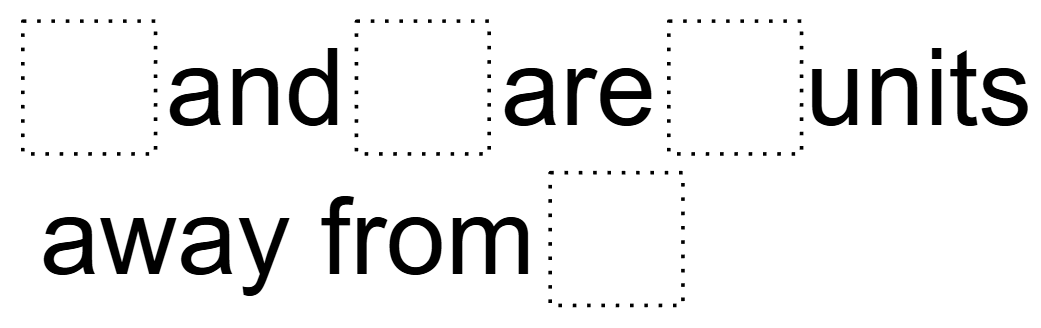# Absolute Value

Directions: Using the digits 1 to 9 at most one time each, fill in the boxes to make the statement true:### Hint

What does absolute value mean? How does that relate to two numbers?

There are many possible solutions. One example would be 3 and 7 are 2 units away from 5

Source: Bryan Anderson

## Two Step Inequality with Fractional Coeffcient

Directions: Using the digits 1 to 7 at most one time each, place a digit …

1.I found 3 and 5 are 1 unit away from 9

•That isn’t correct. 3 and 5 are 1 unit away from 4.

2.I found that 8 and 4 are 2 units away from 6.

3.1 and 7 are 3 units from 4
1 and 9 are 4 units from 5